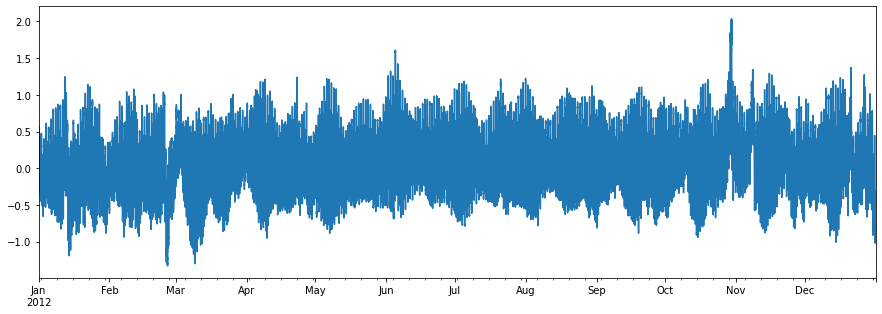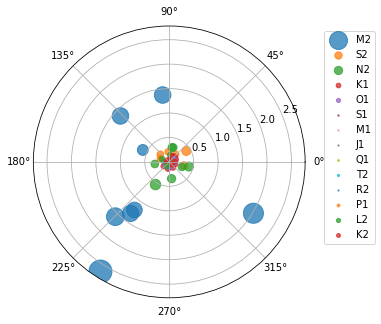This page was generated from noaa.ipynb. Interactive online version:# Water Levels and Tides#

:

from pathlib import Path

import matplotlib.pyplot as plt
import pandas as pd

import async_retriever as ar


We can get water level recordings of a NOAA’s water level station through the CO-OPS API, Let’s get the data for 8534720 station (Atlantic City, NJ), during 2012,. Note that this CO-OPS product has a 31-day for a single request, so we have to break the request accordingly.

:

station_id = "8534720"
start = pd.to_datetime("2012-01-01")
end = pd.to_datetime("2012-12-31")

s = start
dates = []
for e in pd.date_range(start, end, freq="m"):
dates.append((s.date(), e.date()))
s = e + pd.offsets.MonthBegin()

url = "https://api.tidesandcurrents.noaa.gov/api/prod/datagetter"

urls, kwds = zip(
*(
(
url,
{
"params": {
"product": "water_level",
"application": "web_services",
"begin_date": f'{s.strftime("%Y%m%d")}',
"end_date": f'{e.strftime("%Y%m%d")}',
"datum": "MSL",
"station": f"{station_id}",
"time_zone": "GMT",
"units": "metric",
"format": "json",
}
},
)
for s, e in dates
)
)

resp = ar.retrieve(urls, "json", request_kwds=kwds)
wl_list = []
for rjson in resp:
wl = pd.DataFrame.from_dict(rjson["data"])
wl["t"] = pd.to_datetime(wl.t)
wl = wl.set_index(wl.t).drop(columns="t")
wl["v"] = pd.to_numeric(wl.v, errors="coerce")
wl_list.append(wl)
water_level = pd.concat(wl_list).sort_index()

:

ax = water_level.v.plot(figsize=(15, 5))
ax.set_xlabel("")
ax.figure.savefig("_static/water_level.png", bbox_inches="tight", facecolor="w")Now, let’s see an example without any payload or headers. Here’s how we can retrieve harmonic constituents from CO-OPS:

:

stations = [
"8410140",
"8411060",
"8413320",
"8418150",
"8419317",
"8419870",
"8443970",
"8447386",
]

base_url = "https://api.tidesandcurrents.noaa.gov/mdapi/prod/webapi/stations"
urls = [f"{base_url}/{i}/harcon.json?units=metric" for i in stations]
resp = ar.retrieve(urls, "json")

amp_list = []
phs_list = []
for rjson in resp:
sid = rjson["self"].rsplit("/", 2)
const = pd.DataFrame.from_dict(rjson["HarmonicConstituents"]).set_index("name")
amp = const.rename(columns={"amplitude": sid})[sid]
phase = const.rename(columns={"phase_GMT": sid})[sid]
amp_list.append(amp)
phs_list.append(phase)

amp = pd.concat(amp_list, axis=1)
phs = pd.concat(phs_list, axis=1)

:

const = [
"M2",
"S2",
"N2",
"K1",
"O1",
"S1",
"M1",
"J1",
"Q1",
"T2",
"R2",
"P1",
"L2",
"K2",
]

ax = plt.subplot(111, projection="polar")
ax.figure.set_size_inches(5, 5)
for i in const:
phs_ord = phs.loc[i].sort_values()
amp_ord = amp.loc[i][phs_ord.index]
ax.scatter(phs_ord, amp_ord, s=amp_ord * 200, cmap="hsv", alpha=0.75, label=i)

ax.legend(loc="upper left", bbox_to_anchor=(1.05, 1))
ax.figure.savefig(Path("_static", "tides.png"), bbox_inches="tight", facecolor="w")